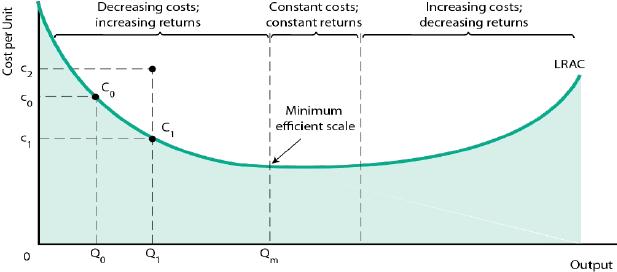Textbook Notes (280,000)
CA (170,000)
McGill (5,000)
MUAR (2)
. (30)
Chapter 8

# MUAR 211 Chapter 8: Chapter 8.docx

Department
Music - Arts
Course Code
MUAR 211
Professor
.
Chapter
8

This preview shows page 1. to view the full 4 pages of the document.Chapter 8: Producers in the Long Run
8.1 The Long Run: No Fixed Factors
In the long run, all inputs are variable.
Firms strive for both technical efficiency and economic efficiency.
Profit Maximization and cost minimization
For any level of output, maximizing profits requires firms to choose their inputs to minimize total
costs.
Using K and L to represent capital and labour, and PL and PK as the prices for the two factors,
cost is minimized when: MPK/PK=MPL/PL or MPK/MPL= PK/PL
*If you want to produce given amount of output, you need to look at the combination at capital
and labor so that this equation holds true. What is the level of labour and what is the level of
capital and how to make those equal?*. To maximize profit. Your technology allows to replace
workers or capital.
The principle of substitution: firms adjust the quantities of factors in response to changing
relative factor prices.
Examples of the principle of substitution in action:
1. Methods of producing the same product often differ across countries
2. Airplanes using more fuel efficient technologies.
3. The use of electricity across different countries.
Long run cost curves
When all factors of production can be varied, consider the least-cost method of producing any
level of output.
The long run average cost (LRAC) curve separates unattainable
and attainable cost levels, given technology and factor prices.
The LRAC curve is usually U-shaped.
Fig. 8-11 A “saucer shaped” Long run average cost Curve